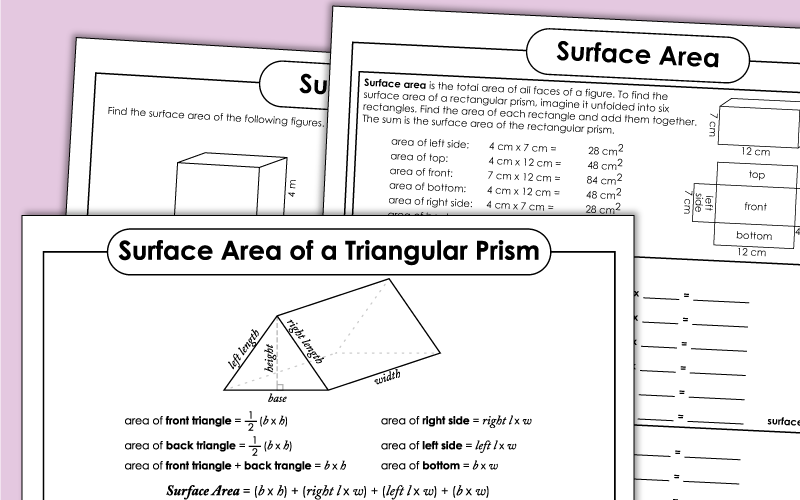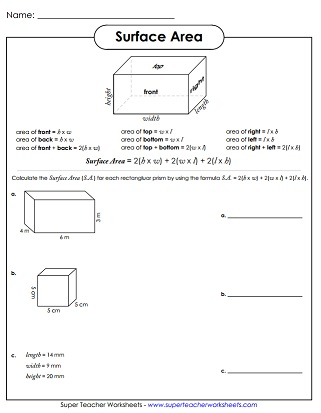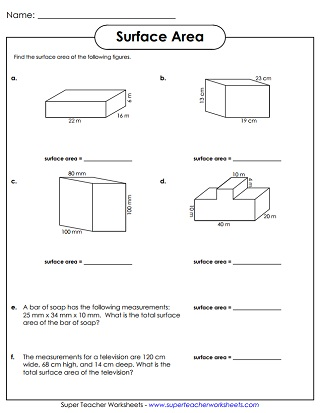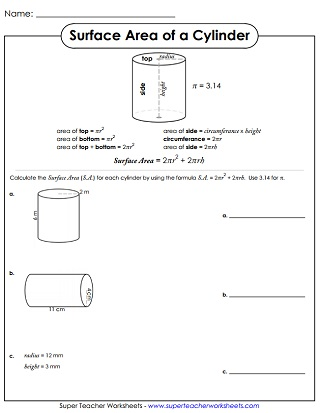# Surface Area Worksheets

This page has worksheets for calculating the area of rectangular prisms and other solid shapes.## Level: Basic(Rectangular Prisms &Cubes Only)

This worksheet has an explanatory box to show students how to solve surface area problems. Then there are asked to find the SA for three different rectangular prisms.
Learn about surface area by folding paper to make a 3D model of a rectangular prism. Count the squares on the sides to find the SA.
Practice calculating S.A. using the mathematical formula SA = 2(h x w) + 2(w x l) + 2(l x h).
Calculate the surface area of the three rectangular prisms shown. Show your work in the blank space to the right of each problem.
This worksheet has three solid shapes, with the dimensions labeled. Students determine the SA of each. Includes plenty of space to show work.

## Level: Intermediate

Find the surface area of two rectangular prisms and an irregular solid shape.
Calculate the surface area of three solid shapes. This worksheet includes space for students to show their work.
This printable activity has four graphical shapes. Pupils calculate the surface area for each. Then they solve two word problems.

To calculate the S.A. of a cylinder, students must know the height and radius. Using the given values, find the S.A. for three different cylindrical shapes.
Using the given formula, figure out the SA of three triangular prisms.
Use the given formula to determine the surface area of the spheres. Includes a sample, plus three spheres to solve.
Area Worksheets

Find the area of squares, rectangles, triangles, and circles. Includes basic, intermediate, and advanced-level worksheets.

Order of Operations

Learn to solve operations in order: parenthesis, exponents, multiply & divide, add & subtract.

## Sample Worksheet ImagesMy Account
Site Information